|

# 【欣赏】国外那些唯美中式建筑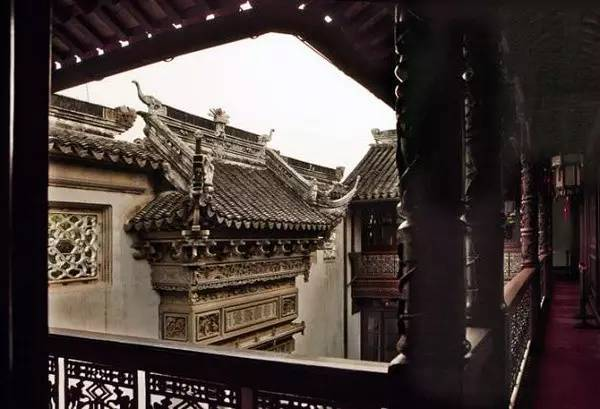17至18世纪在欧洲文化史上有一个词语颇为引人注意，这就是chinoiserie（中国风）。当时中国的瓷器、壁纸、刺绣、服装、家具、建筑等风靡了以英国和法国为代表的欧洲国家。其中特别重要的是中国的园林，它深刻影响了欧洲的造园艺术，使之发生了巨大的变化。短短几十年间，欧洲大陆上兴建了不少中式建筑。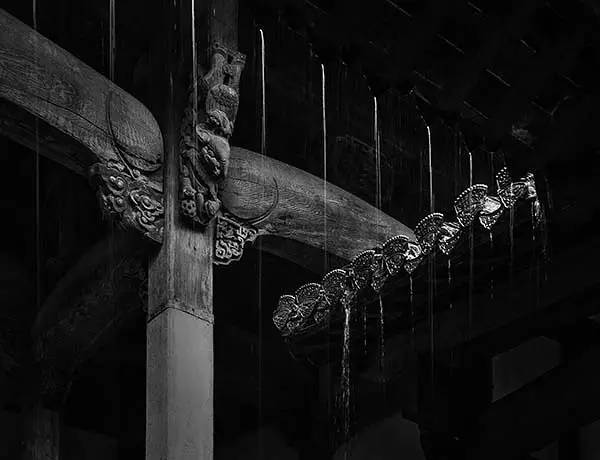18世纪的欧洲花园多为庄园府邸的附属花园，供主人游览观赏之用，多数为私家，如瑞典的“中国宫”，法国的“红楼”。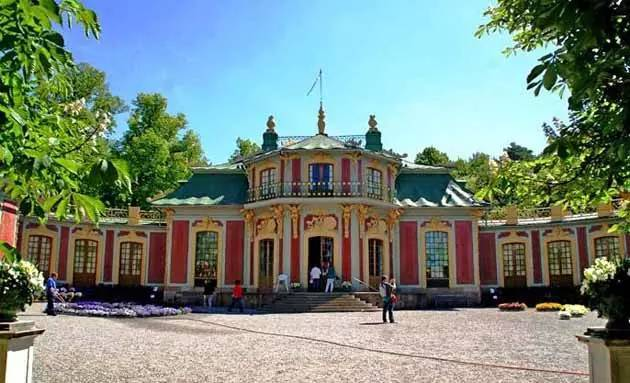瑞典“中国宫”： 位于斯德哥尔摩市郊的皇后岛上。由于王后乌尔利卡非常喜爱中国文化，1753年，国王腓特烈一世在离王宫不远的地方专门为她修建了中国式楼阁。这是一座中国式宫殿与法国洛可可式建筑的融合体，整个建筑呈弓形，宫顶仿中国宫殿装饰并刻意雕龙，但无琉璃瓦。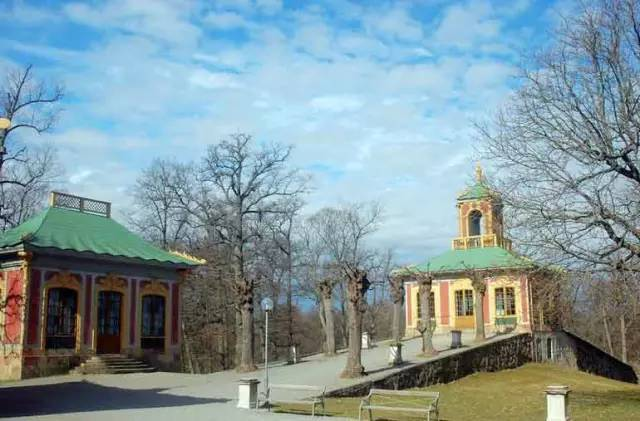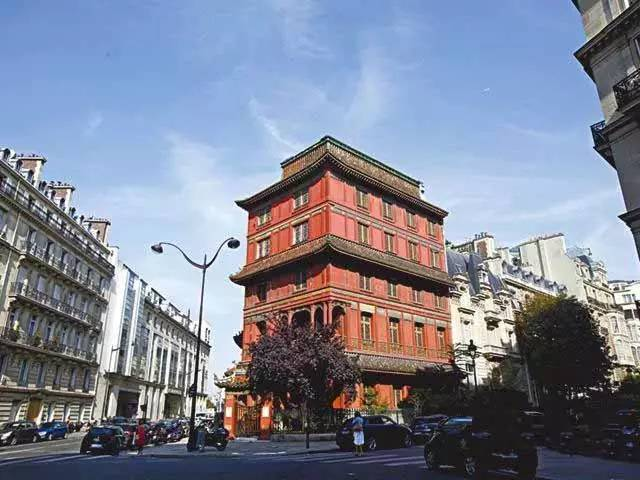法国红楼：位于巴黎富人区蒙梭公园附近一座赭红色的中式楼房，这是巴黎城内极少出现与城市整体风格不一致的建筑。建造者卢芹斋，是19世纪末来到法国的中国人。这座中式建筑，最初使命原本是装载中国古董，但是随着时间的推移，其建筑本身已经成为进入巴黎文化遗产之列的古董。

18世纪欧洲的中国园林，在很大程度上融合了中国园林和西方园林的特色。由于部分花园是从古典式改建而来，还在局部保留了古典主义的手法，东西合璧的做法比较多见。总的来说，这时的欧洲中国园林，多数是在局部模仿，手法比较简单。自然风致园相对中国传统园林而言，处理过于粗糙，类似荒野的景色，缺乏中国园林的精心布置。图画式园在此基础上增加了一些中国建筑，不过模仿得不太地道。比如德国慕尼黑英国公园里的“中国塔”。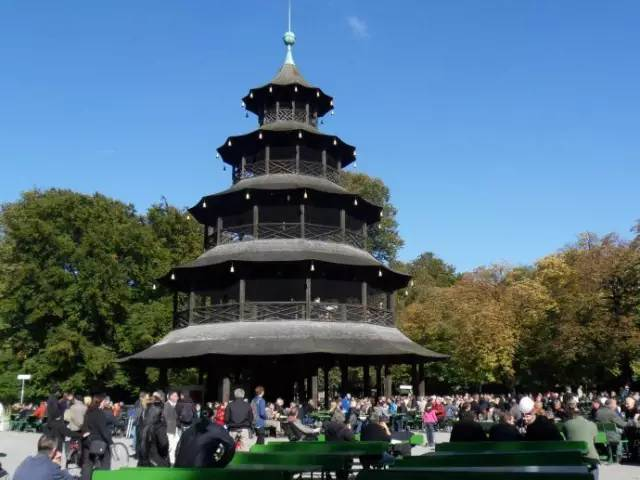慕尼黑英国公园里的“中国塔”（Chinesischer Turm）。

这是德国人按照自己对中国木塔的想象建成的塔，美名曰“中国塔”。中国塔是1789-1790年建造的，设计和制作全凭着一张画完成的，没有细致地考证真正中国建造的塔的每个细节，所以造出来的中国塔对中国人来说，似乎有点不大像。那时的德国人向往遥远的东方艺术，却没能长途跋涉来到中国，于是半凭传说、半凭想象造出了这样一座塔。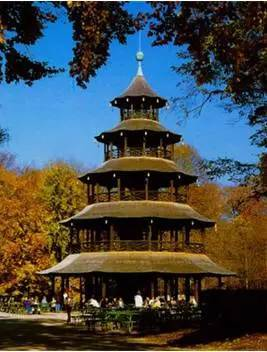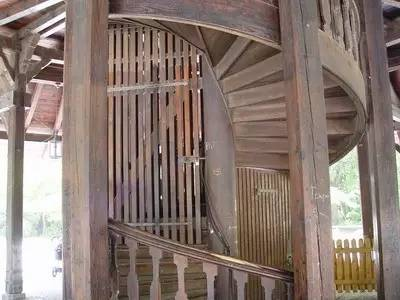18世纪之后，欧洲的“中国热”渐渐消退，以后的一两百年间，中国一直处于被侵略、鄙视的地位，中国文化的价值呈现出弱势的状态。西方人直到20世纪80年代才再次在自己国家见到中国式园林。1980年后修建的中国式园林内容更为丰富，大致有以下几种：展览厅、园艺节参展作品、城市之间的友好赠建、纪念性的修建、观光园林。

纽约大都会博物馆 明轩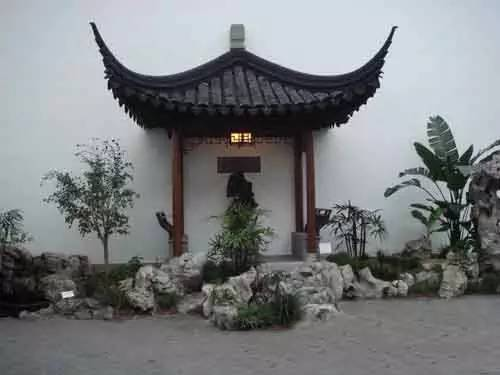慕尼黑  芳华园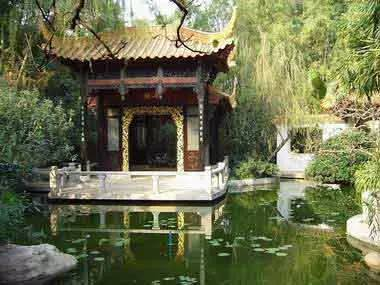蒙特利尔  梦湖园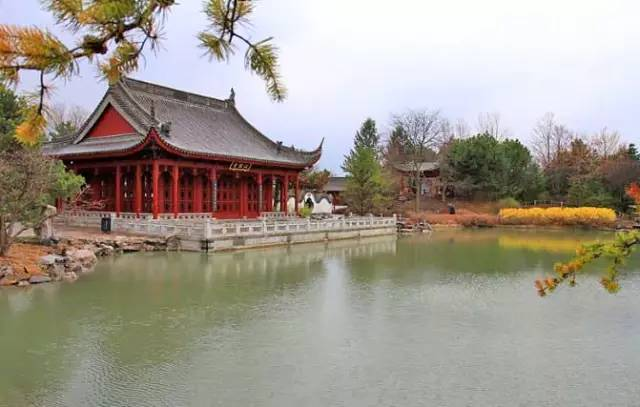瑞士苏黎世市  中国园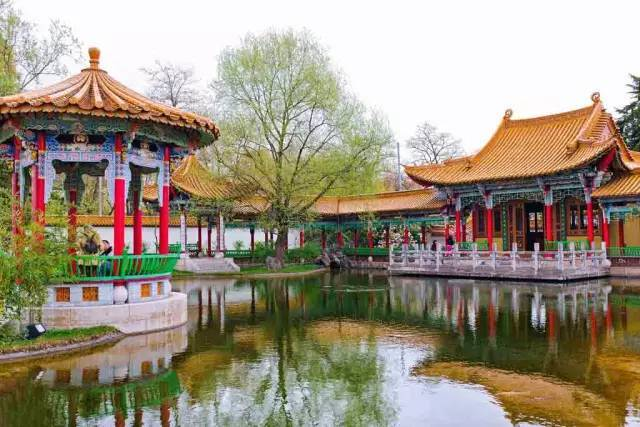中国园于1994年正式开放，是苏黎世的姐妹城市——昆明所赠，昆明市政府仿翠湖公园的格局设计，是海外最大规模的中国式园林。苏黎世人为他们的城市与昆明的友城关系而深深自豪。迄今为止，苏黎世只同昆明保持着唯一的友好城市关系。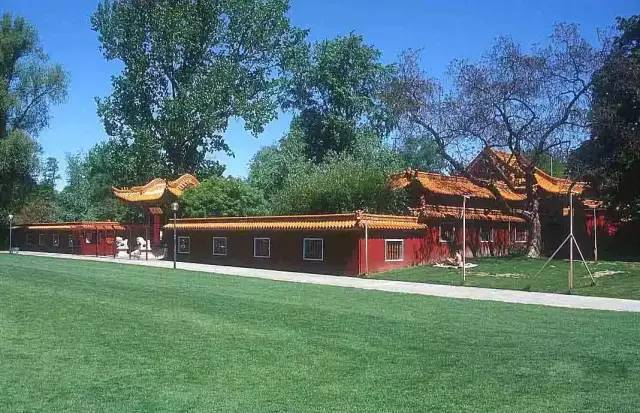新加坡  蕴秀园“蕴秀园”除苏州式的景观盆景之外，亦采用中国岭南派、川派、杨派、苏派和海派设计的盆景。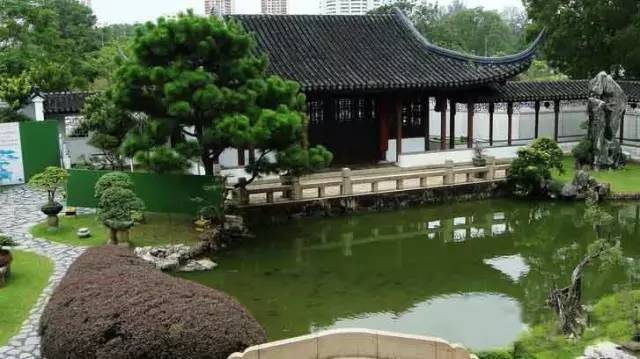法兰克福  春华园纽约斯坦顿岛  寄兴园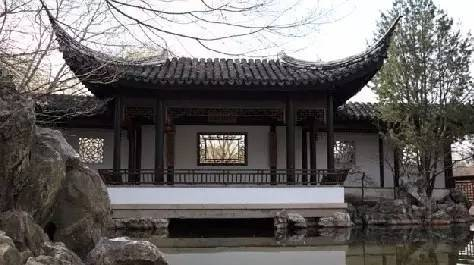德国  郢趣园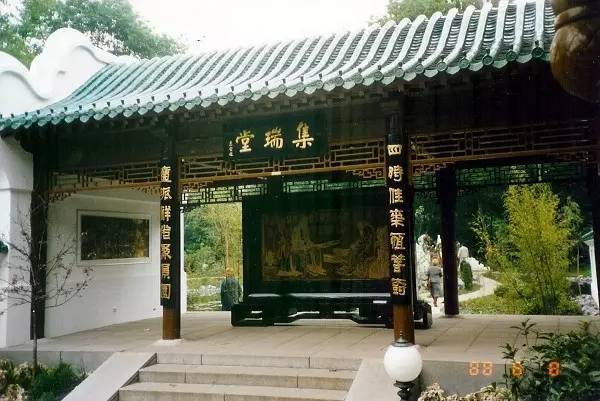德国  豫园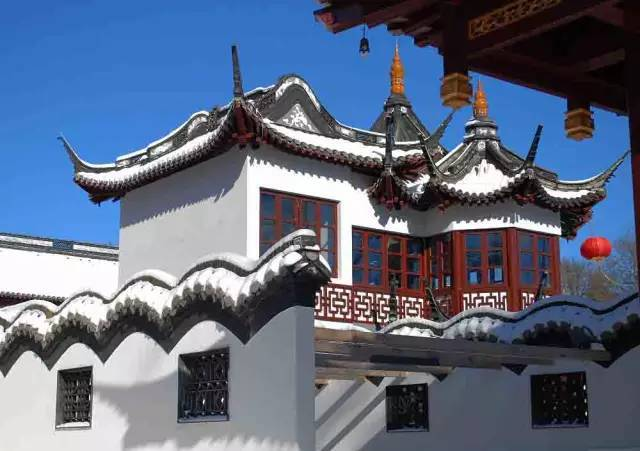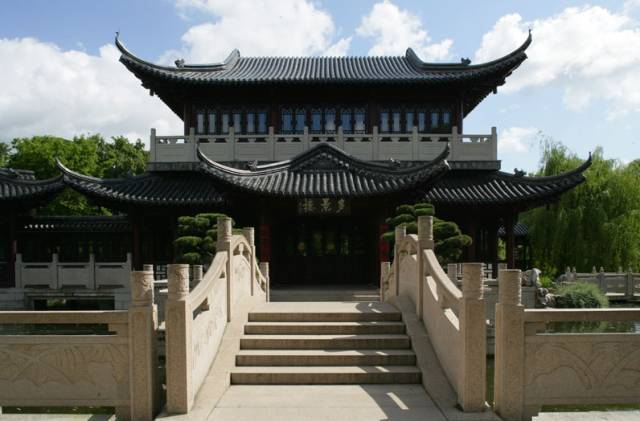日本熊本县  孔子公园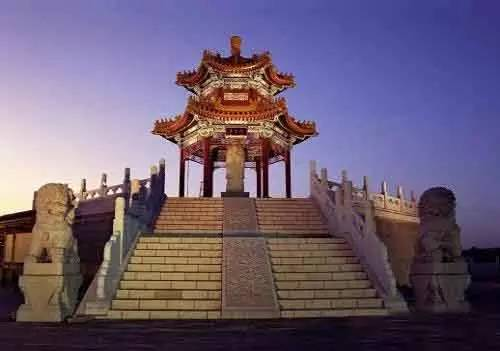英国  燕秀园“燕秀”题名喻其风格具有我国宫廷园林的神韵，园中的建筑是仿照北京北海公园静心斋建造的。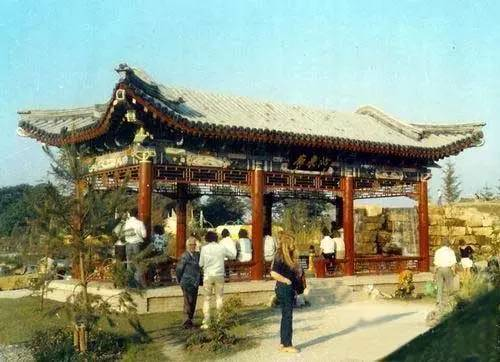日本横滨  友谊园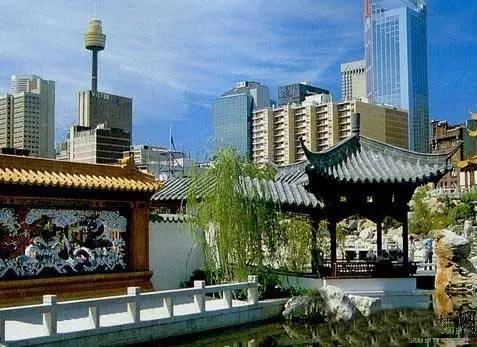美国西雅图  西华园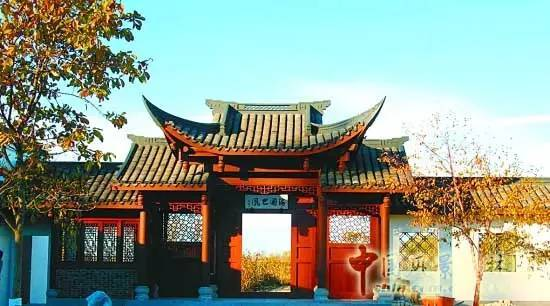加拿大温哥华  逸园澳大利亚悉尼  谊园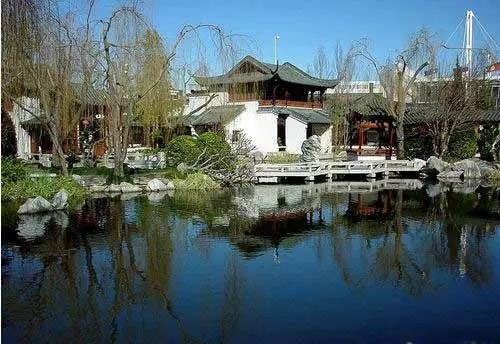`声明：本文由入驻焦点开放平台的作者撰写，除焦点官方账号外，观点仅代表作者本人，不代表焦点立场错误信息举报电话： 400-099-0099，邮箱：jubao@vip.sohu.com，或点此进行意见反馈，或点此进行举报投诉。`A B C D E F G H J K L M N P Q R S T W X Y Z
A - B - C - D - E
• A
• 鞍山
• 安庆
• 安阳
• 安顺
• 安康
• 澳门
• B
• 北京
• 保定
• 包头
• 巴彦淖尔
• 本溪
• 蚌埠
• 亳州
• 滨州
• 北海
• 百色
• 巴中
• 毕节
• 保山
• 宝鸡
• 白银
• 巴州
• C
• 承德
• 沧州
• 长治
• 赤峰
• 朝阳
• 长春
• 常州
• 滁州
• 池州
• 长沙
• 常德
• 郴州
• 潮州
• 崇左
• 重庆
• 成都
• 楚雄
• 昌都
• 慈溪
• 常熟
• D
• 大同
• 大连
• 丹东
• 大庆
• 东营
• 德州
• 东莞
• 德阳
• 达州
• 大理
• 德宏
• 定西
• 儋州
• 东平
• E
• 鄂尔多斯
• 鄂州
• 恩施
F - G - H - I - J
• F
• 抚顺
• 阜新
• 阜阳
• 福州
• 抚州
• 佛山
• 防城港
• G
• 赣州
• 广州
• 桂林
• 贵港
• 广元
• 广安
• 贵阳
• 固原
• H
• 邯郸
• 衡水
• 呼和浩特
• 呼伦贝尔
• 葫芦岛
• 哈尔滨
• 黑河
• 淮安
• 杭州
• 湖州
• 合肥
• 淮南
• 淮北
• 黄山
• 菏泽
• 鹤壁
• 黄石
• 黄冈
• 衡阳
• 怀化
• 惠州
• 河源
• 贺州
• 河池
• 海口
• 红河
• 汉中
• 海东
• 怀来
• I
• J
• 晋中
• 锦州
• 吉林
• 鸡西
• 佳木斯
• 嘉兴
• 金华
• 景德镇
• 九江
• 吉安
• 济南
• 济宁
• 焦作
• 荆门
• 荆州
• 江门
• 揭阳
• 金昌
• 酒泉
• 嘉峪关
K - L - M - N - P
• K
• 开封
• 昆明
• 昆山
• L
• 廊坊
• 临汾
• 辽阳
• 连云港
• 丽水
• 六安
• 龙岩
• 莱芜
• 临沂
• 聊城
• 洛阳
• 漯河
• 娄底
• 柳州
• 来宾
• 泸州
• 乐山
• 六盘水
• 丽江
• 临沧
• 拉萨
• 林芝
• 兰州
• 陇南
• M
• 牡丹江
• 马鞍山
• 茂名
• 梅州
• 绵阳
• 眉山
• N
• 南京
• 南通
• 宁波
• 南平
• 宁德
• 南昌
• 南阳
• 南宁
• 内江
• 南充
• P
• 盘锦
• 莆田
• 平顶山
• 濮阳
• 攀枝花
• 普洱
• 平凉
Q - R - S - T - W
• Q
• 秦皇岛
• 齐齐哈尔
• 衢州
• 泉州
• 青岛
• 清远
• 钦州
• 黔南
• 曲靖
• 庆阳
• R
• 日照
• 日喀则
• S
• 石家庄
• 沈阳
• 双鸭山
• 绥化
• 上海
• 苏州
• 宿迁
• 绍兴
• 宿州
• 三明
• 上饶
• 三门峡
• 商丘
• 十堰
• 随州
• 邵阳
• 韶关
• 深圳
• 汕头
• 汕尾
• 三亚
• 三沙
• 遂宁
• 山南
• 商洛
• 石嘴山
• T
• 天津
• 唐山
• 太原
• 通辽
• 铁岭
• 泰州
• 台州
• 铜陵
• 泰安
• 铜仁
• 铜川
• 天水
• 天门
• W
• 乌海
• 乌兰察布
• 无锡
• 温州
• 芜湖
• 潍坊
• 威海
• 武汉
• 梧州
• 渭南
• 武威
• 吴忠
• 乌鲁木齐
X - Y - Z
• X
• 邢台
• 徐州
• 宣城
• 厦门
• 新乡
• 许昌
• 信阳
• 襄阳
• 孝感
• 咸宁
• 湘潭
• 湘西
• 西双版纳
• 西安
• 咸阳
• 西宁
• 仙桃
• 西昌
• Y
• 运城
• 营口
• 盐城
• 扬州
• 鹰潭
• 宜春
• 烟台
• 宜昌
• 岳阳
• 益阳
• 永州
• 阳江
• 云浮
• 玉林
• 宜宾
• 雅安
• 玉溪
• 延安
• 榆林
• 银川
• Z
• 张家口
• 镇江
• 舟山
• 漳州
• 淄博
• 枣庄
• 郑州
• 周口
• 驻马店
• 株洲
• 张家界
• 珠海
• 湛江
• 肇庆
• 中山
• 自贡
• 资阳
• 遵义
• 昭通
• 张掖
• 中卫

1室1厅1厨1卫1阳台

1
2
3
4
5

0
1
2

1

1

0
1
2
3报名成功，资料已提交审核A B C D E F G H J K L M N P Q R S T W X Y Z
A - B - C - D - E
• A
• 鞍山
• 安庆
• 安阳
• 安顺
• 安康
• 澳门
• B
• 北京
• 保定
• 包头
• 巴彦淖尔
• 本溪
• 蚌埠
• 亳州
• 滨州
• 北海
• 百色
• 巴中
• 毕节
• 保山
• 宝鸡
• 白银
• 巴州
• C
• 承德
• 沧州
• 长治
• 赤峰
• 朝阳
• 长春
• 常州
• 滁州
• 池州
• 长沙
• 常德
• 郴州
• 潮州
• 崇左
• 重庆
• 成都
• 楚雄
• 昌都
• 慈溪
• 常熟
• D
• 大同
• 大连
• 丹东
• 大庆
• 东营
• 德州
• 东莞
• 德阳
• 达州
• 大理
• 德宏
• 定西
• 儋州
• 东平
• E
• 鄂尔多斯
• 鄂州
• 恩施
F - G - H - I - J
• F
• 抚顺
• 阜新
• 阜阳
• 福州
• 抚州
• 佛山
• 防城港
• G
• 赣州
• 广州
• 桂林
• 贵港
• 广元
• 广安
• 贵阳
• 固原
• H
• 邯郸
• 衡水
• 呼和浩特
• 呼伦贝尔
• 葫芦岛
• 哈尔滨
• 黑河
• 淮安
• 杭州
• 湖州
• 合肥
• 淮南
• 淮北
• 黄山
• 菏泽
• 鹤壁
• 黄石
• 黄冈
• 衡阳
• 怀化
• 惠州
• 河源
• 贺州
• 河池
• 海口
• 红河
• 汉中
• 海东
• 怀来
• I
• J
• 晋中
• 锦州
• 吉林
• 鸡西
• 佳木斯
• 嘉兴
• 金华
• 景德镇
• 九江
• 吉安
• 济南
• 济宁
• 焦作
• 荆门
• 荆州
• 江门
• 揭阳
• 金昌
• 酒泉
• 嘉峪关
K - L - M - N - P
• K
• 开封
• 昆明
• 昆山
• L
• 廊坊
• 临汾
• 辽阳
• 连云港
• 丽水
• 六安
• 龙岩
• 莱芜
• 临沂
• 聊城
• 洛阳
• 漯河
• 娄底
• 柳州
• 来宾
• 泸州
• 乐山
• 六盘水
• 丽江
• 临沧
• 拉萨
• 林芝
• 兰州
• 陇南
• M
• 牡丹江
• 马鞍山
• 茂名
• 梅州
• 绵阳
• 眉山
• N
• 南京
• 南通
• 宁波
• 南平
• 宁德
• 南昌
• 南阳
• 南宁
• 内江
• 南充
• P
• 盘锦
• 莆田
• 平顶山
• 濮阳
• 攀枝花
• 普洱
• 平凉
Q - R - S - T - W
• Q
• 秦皇岛
• 齐齐哈尔
• 衢州
• 泉州
• 青岛
• 清远
• 钦州
• 黔南
• 曲靖
• 庆阳
• R
• 日照
• 日喀则
• S
• 石家庄
• 沈阳
• 双鸭山
• 绥化
• 上海
• 苏州
• 宿迁
• 绍兴
• 宿州
• 三明
• 上饶
• 三门峡
• 商丘
• 十堰
• 随州
• 邵阳
• 韶关
• 深圳
• 汕头
• 汕尾
• 三亚
• 三沙
• 遂宁
• 山南
• 商洛
• 石嘴山
• T
• 天津
• 唐山
• 太原
• 通辽
• 铁岭
• 泰州
• 台州
• 铜陵
• 泰安
• 铜仁
• 铜川
• 天水
• 天门
• W
• 乌海
• 乌兰察布
• 无锡
• 温州
• 芜湖
• 潍坊
• 威海
• 武汉
• 梧州
• 渭南
• 武威
• 吴忠
• 乌鲁木齐
X - Y - Z
• X
• 邢台
• 徐州
• 宣城
• 厦门
• 新乡
• 许昌
• 信阳
• 襄阳
• 孝感
• 咸宁
• 湘潭
• 湘西
• 西双版纳
• 西安
• 咸阳
• 西宁
• 仙桃
• 西昌
• Y
• 运城
• 营口
• 盐城
• 扬州
• 鹰潭
• 宜春
• 烟台
• 宜昌
• 岳阳
• 益阳
• 永州
• 阳江
• 云浮
• 玉林
• 宜宾
• 雅安
• 玉溪
• 延安
• 榆林
• 银川
• Z
• 张家口
• 镇江
• 舟山
• 漳州
• 淄博
• 枣庄
• 郑州
• 周口
• 驻马店
• 株洲
• 张家界
• 珠海
• 湛江
• 肇庆
• 中山
• 自贡
• 资阳
• 遵义
• 昭通
• 张掖
• 中卫• 手机• 分享
• 设计
免费设计
• 计算器
装修计算器
• 入驻
合作入驻
• 联系
联系我们
• 置顶
返回顶部